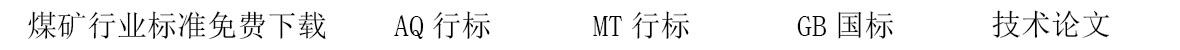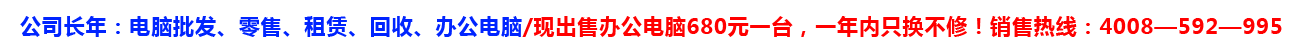# 井巷工程各种断面计算公式

##### 下载地址

S=B·h
L=B＋2h=2(B＋h)

S=(a＋b)/2·h
L=4.16S=B·（h＋0.26B）= B·h＋0.26B2
S全高计算=BH－0.293B
L=2.93B＋2h
L=4.1S=B·（h＋0.39B）=Bh＋0.39B2
S=B·h＋π.B2/8
S全高计算=BH－0.108B2
L=3.84L=2.57B＋2h=3B＋π.B/2

S=π.D2/4
S=π.r2
L=π.D=2π.r

S=a2,正方形面积=边长×边长

S=a×b 长方形面积=长×宽

##### 版权声明

1.本站遵循行业规范，任何转载的稿件都会明确标注作者和来源；

2.本站的原创文章，请转载时务必注明文章作者和来源，不尊重原创的行为我们将追究责任；3.作者投稿可能会经我们编辑修改或补充。